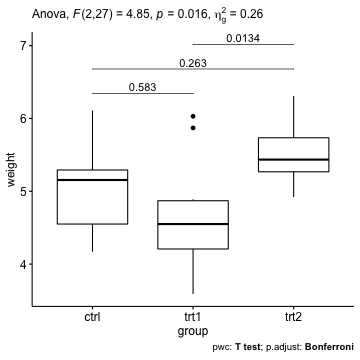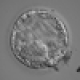# How to Perform T-test for Multiple Groups in R## Prerequisites

# Load required R packages
library(tidyverse)
library(rstatix)
library(ggpubr)

# Prepare the data and inspect a random sample of the data
data("PlantGrowth")
set.seed(1234)
PlantGrowth %>% sample_n_by(group, size = 1)
## # A tibble: 3 x 2
##   weight group
##    <dbl> <fct>
## 1   5.58 ctrl
## 2   6.03 trt1
## 3   4.92 trt2

## Summary statistics

PlantGrowth %>%
group_by(group) %>%
get_summary_stats(weight, type = "mean_sd")
## # A tibble: 3 x 5
##   group variable     n  mean    sd
##   <fct> <chr>    <dbl> <dbl> <dbl>
## 1 ctrl  weight      10  5.03 0.583
## 2 trt1  weight      10  4.66 0.794
## 3 trt2  weight      10  5.53 0.443

## Compare the mean of multiple groups using ANOVA test

res.aov <- PlantGrowth %>% anova_test(weight ~ group)
res.aov
## ANOVA Table (type II tests)
##
##   Effect DFn DFd    F     p p<.05   ges
## 1  group   2  27 4.85 0.016     * 0.264

From the above ANOVA table, it can be seen that there are significant differences between groups (p = 0.016), which are highlighted with “*“, F(2, 27) = 4.85, p = 0.016, eta2[g] = 0.26.

## Pairwise T-tests for multiple groups

# Pairwise comparisons
pwc <- PlantGrowth %>%
pairwise_t_test(weight ~ group, p.adjust.method = "bonferroni")
pwc
## # A tibble: 3 x 9
## * <chr>  <chr>  <chr>  <int> <int>   <dbl> <chr>     <dbl> <chr>
## 1 weight ctrl   trt1      10    10 0.194   ns       0.583  ns
## 2 weight ctrl   trt2      10    10 0.0877  ns       0.263  ns
## 3 weight trt1   trt2      10    10 0.00446 **       0.0134 *

## Visualization: box plots with p-values

# Show adjusted p-values
pwc <- pwc %>% add_xy_position(x = "group")
ggboxplot(PlantGrowth, x = "group", y = "weight") +
stat_pvalue_manual(pwc, label = "p.adj", tip.length = 0, step.increase = 0.1) +
labs(
subtitle = get_test_label(res.aov, detailed = TRUE),
caption = get_pwc_label(pwc)
)# Show significance levels
# Hide non-significant tests
ggboxplot(PlantGrowth, x = "group", y = "weight") +
stat_pvalue_manual(pwc, hide.ns = TRUE, label = "p.adj.signif") +
labs(
subtitle = get_test_label(res.aov, detailed = TRUE),
caption = get_pwc_label(pwc)
)### Comment ( 1 )

•Fredy Mejía

THANK YOU!